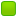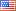Author Topic: I have a problem with a simple 64bit programm  (Read 532 times)Renegade

• Jr. Member
•• Posts: 2I have a problem with a simple 64bit programm
« on: February 06, 2019, 02:03:16 AM »
Hey guys,
I wrote a code to search for primenumbers in a given range.
My programm shall take an argument, which will be the last number checked.
To accelerate the code i wanna check divisibility through 2,3,5,7 before i increase the divisor.
If the divisor reaches the current number x/2 you can be sure that it is prime.

I am sorry bothering here for help, but i don't understand whats wrong with my code, because it gives my an errormessage while linking.
Also do i want to find large primenumbers > 1000000 etc....
Therefore i am not sure if i declared the size of "output" correctly.

Code: [Select]

; Compiled with
; nasm -f elf64 prime.asm && gcc -o prime prime.o

global _start
extern atoi
extern printf
extern puts

section .text

main:
cmp rdi, 2 ;check if it has one argument
jne error1
xor rdi, rdi

mov rdi, rsi; argument
call atoi wrt ..plt
mov r8, rdi
inc r8
xor rdi, rdi
mov r9, 2 ;number to begin
mov r11, 11

checkPrime2: ; check if divisible by 2
xor rdx, rdx
cmp r8, r9
je done
mov rax, r9
mov rcx, 2
div rcx
mov r10, rax
cmp rdx, 0
je notPrime

checkPrime3: ; check if divisible by 3
xor rdx, rdx
mov rax, r9
mov rcx, 3
div rcx
cmp rdx, 0
je notPrime

checkPrime5: ; check if divisibly by 5
xor rdx, rdx
mov rax, r9
mov rcx, 5
div rcx
cmp rdx, 0
je notPrime

checkPrime7: ; check if is divisible by 7
xor rdx, rdx
mov rax, r9
mov rcx, 7
div rcx
cmp rdx, 0
je notPrime

; increase divisor until it reaches half of the given number.
; r11 is the divisor which will be incremented
; in r10 is the actual number divided by two
divisorIncrease:
xor rcx, rcx
mov rax, r9
mov rcx, r11
div rcx
cmp rdx, 0
je notPrime
cmp r11, r10
je printPrime
inc r11
jmp divisorIncrease

printPrime:
mov rdi, output
mov rsi, r9
call printf wrt ..plt
inc r9
cmp r8, r9 ; r8 contains the argument (highest number) +1
je done
jmp checkPrime2

notPrime:
inc r9
cmp r8, r9
je done
jmp checkPrime2

error1:
mov rdi, noArgument
call puts wrt ..plt

done:
ret

section .data

output:
dq "%d", 10, 0

noArgument:
db "Requires exactly one argument (last number to check)", 10, 0

I try to compile and link this code to make an executable, but everytime i get this error:

nasm -f elf64 prime.asm && gcc -static -m64 prime.o -o prime
/usr/bin/ld: /usr/lib/gcc/x86_64-linux-gnu/8/../../../x86_64-linux-gnu/crt1.o: in function `_start':
(.text+0x20): undefined reference to `main'
collect2: error: ld returned 1 exit status

Best Regards and very gracefully,
RenegadeFrank Kotler

• NASM Developer
• Hero Member
•• Posts: 2379
• Country:Re: I have a problem with a simple 64bit programm
« Reply #1 on: February 06, 2019, 02:37:42 AM »

Welcome to the Forum!

To start with, replace "global _start" with "global main". I think that will make it assemble and link. There may be other issues.

Best,
Frankfredericopissarra

• Jr. Member
•• Posts: 53Re: I have a problem with a simple 64bit programm
« Reply #2 on: February 12, 2019, 07:20:00 PM »
Also, this is wrong:

Code: [Select]
mov  rdi, rsi; argument
call atoi wrt ..plt

The 2nd argument of main() is a pointer to an array of pointers. The correct reference is:

Code: [Select]
mov rdi,[rsi+8]
And atoi() isn't a libc function, but a macro for strtol().Renegade

• Jr. Member
•• Posts: 2Re: I have a problem with a simple 64bit programm
« Reply #3 on: February 15, 2019, 12:18:31 AM »
Yo guyz,
I rewrote the code.
And it's running very good !
Check this out.Code: [Select]

;compiled with
;nasm -f elf64 prime64.asm && gcc -static -O3 prime64.o -o prime64

global main
extern printf
extern puts
extern atoi

section .text

main:
push r12 ;free r12
push r13 ;free r13
push r14 ;free r14

cmp rdi, 2 ; check if program+one arguments are given
jne error1 ; when rdi is not 3 jump to error1

mov r12, rsi ; mov argv to r12
mov rdi, [r12+8] ; mov argv (number) in rdi
call atoi WRT ..plt ; convert to integer (returns to eax)
cmp eax, 2 ; compare it to 0
jl error2 ; if less than 0 jmp to error2
inc eax
mov r13d, eax ; biggest number
mov r12d, 2 ; divisor
xor r11d, 0

case2:
mov eax, 2 ; dont check if two is prime ;)
jmp first

primecheck2:
inc r14d ;actual number is in r14d
xor edx, edx ;f*** that we exclude divisibles trough 2 directly
mov eax, r14d
mov ecx, 2
div ecx
cmp edx, 0
je notprime
mov r12d, 2 ; r12d is the register which holds the divisor

primeloop:
xor edx, edx
inc r12d ; increment the divisor
cmp r12d, r14d ; is our number the divisor ? --> prime
je isprime
mov eax, r14d
mov ecx, r12d
div ecx
cmp edx, 0 ; we check remainder if its zero ? true == notprime
je notprime
jmp primeloop ; loop again

notprime:
cmp r14d, r13d ; are  we done ?
jl primecheck2 ; if not test next number
jmp done
isprime:
mov eax, r14d ; first time we print 2 directly (yeah it looks ugly) :(
first:
mov rdi, answer1 ; write ansswer to the rdi
movsxd rsi, eax ; mov eax with sign extension to rsi (conversion to 64 bit number)
mov r14d, eax ; r14d contains the actual number to check
xor rax, rax ; xor a register to itself sets it to 0
call printf WRT ..plt ; call printf
cmp r14d, r13d
jl primecheck2
jmp done

error1:
call puts WRT ..plt ; print out badArgumentCount
jmp done

error2:
mov edi, numberUnderTwo ; the lowest prime number is 2 !
call puts WRT ..plt

done:
pop r14 ; take the values of these register from
pop r13 ; the stack to set them, how they were before
pop r12 ; running the proggy
ret ; return

section .data

db "%d", 10,  0
db "Requires exactly one argument!", 10, 0
numberUnderTwo:
db "The Number may not be under 2, because it is the lowest prime !.", 10, 0

Ps: It would be cool to increase the speed, i will do that in future ! I will advance the algorithm.fredericopissarra

• Jr. Member
•• Posts: 53Re: I have a problem with a simple 64bit programm
« Reply #4 on: February 15, 2019, 01:28:35 PM »
Instead of using glibc functions, you can create a standalone asm application. Instead of using atoi and printf, you can create your own atoi and printf routines. The question is how to get argc and argv?

They are pushed on stack by the kernel RSP points to argc and RSP+8 points to argv:

Code: [Select]
\$ nasm -f elf64 -g -o args.o args.asm
\$ ld -o args args.o
\$ gdb -q args
Reading symbols from args...done.
(gdb) l
1   bits  64
2
3   section .text
4
5   global  _start
6
7 _start:
8   xor   edi,edi       ; syscall_exit(0)
9   mov   eax,60
10   syscall
(gdb) b 8
Breakpoint 1 at 0x400080: file args.asm, line 8.
(gdb) r
Starting program: /home/frederico/MyStuff/tmp/args

Breakpoint 1, 0x0000000000400080 in _start ()
(gdb) p *((int *)\$rsp)
\$1 = 1
(gdb) p ((char **)(\$rsp+8))
\$2 = 0x7fffffffdf4f "/home/frederico/MyStuff/tmp/args"
(gdb) p ((char **)(\$rsp+8))
\$3 = 0x0

So, after _start, you can do:

Code: [Select]
mov  ecx,[rsp]  ; ECX = argc
mov  rsi,[rsp+8]  ; RSP = argv
mov  rbx,[rsi+8]  ; RBX = argv

You can ignore argc and test if RBX is zero (NULL), meaning thare is only one argument on the command line:

Code: [Select]
_start:
mov  rsi,[rsp+8]
mov  rbx,[rsi+8]
test rbx,rbx
je   .only_arg0_error
...

***

To speed up the primalty test you can check only odd values lesser of equal to square root of the number.
A good square root routine for integers is this one: https://en.wikipedia.org/wiki/Integer_square_root.

atoi() is simple to implement (if you don't care with input validation): Initializing the result to 0, for each char, get the correspondent value and add to the previous times 10.

To simulate puts() you can use write syscall, writing to stdout (descriptor 1). To write a decimal number on screen it is only a matter of get the reminder of it by 10 and fill an array. Once filled, print the chars backwards.

This way, your code will be glibc free.
« Last Edit: February 15, 2019, 01:34:58 PM by fredericopissarra »